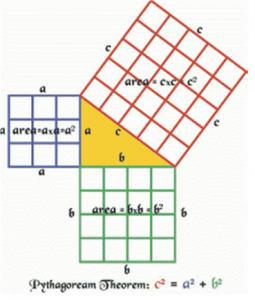# Solving the 2014 APL Problem Solving Competition – It’s All Right

This post is the continuation of the series where we examine some of the problems selected for the 2014 APL Problem Solving Competition.

The problems presented in Phase 1 of the competition were selected because they could be solved succinctly, generally in a single line of APL code. This makes them well suited for experimentation on TryAPL.org.Problem 1 of Phase 1, entitled “It’s all right” reads,

“Write a dfn that takes the length of the legs of a triangle as its left argument, and the length of the hypotenuse as its right argument and returns 1 if the triangle is a right triangle, 0 otherwise.”

```Test cases:```
3 4 {your_solution} 5
1
2 3 {your_solution} 4
0
``````

This uses the Pythagorean theorem – A² + B² = C². It’s trivial to implement an almost direct translation of this in APL – in a dfn, using `⍺` for A, `⍺` for B and `⍵` for C yields:

``right←{((⍺*2)+(⍺*2))=⍵*2}``

This seems rather clunky though… what if we consider the problem as “Are the sums of the squares of each argument equal?” To get the sum of the squares, first we can use the composition `*∘2` (composing the power function `*` with the constant 2) to mean “square” and `+/` to mean “sum”, and combine them in a 2-item function train (also known as an “atop”): `((+/)*∘2)`

then apply this to each argument:   `((+/)*∘2)¨⍺ ⍵`

and compare the results for equality, resulting in the dfn:

``right1←{=/((+/)*∘2)¨⍺ ⍵}``

Still this seems clunky to me. Let’s see…squaring a number is just multiplying the number by itself, so, if we use the monadic commute operator with multiplication,   `×⍨`
we get the equivalent of squaring. Then we can use that function in an inner product with addition to also get “sum of the squares”:   `+.×⍨`

The rest is essentially the same as in `right1` above:

``right2←{=/+.×⍨¨⍺ ⍵}``

All three of these solutions solve the contest problem. The first one, `right`, is not commutative though – the legs of the triangle must be supplied as the left argument. However, `right1` and `right2` are commutative and the order of arguments is not significant.